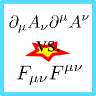Using the useful index notation of special relativity we will find that one may use different Lagrangians to describe relativistic electrodynamics. This problem will make you familiar with the basic rules needed for practical calculations.

## Problem Statement

In some textbooks we find the relativistic Lagrangian of electrodynamics in the form

$\begin{eqnarray*} \mathcal{L}_{1} = -\frac{1}{2\mu_{0}}\partial_{\mu}A_{\nu}\partial^{\mu}A^{\nu}-J_{\mu}A^{\mu}\ .\end{eqnarray*}$

Usually, however, it is given as

$\begin{eqnarray*} \mathcal{L}_{2} = -\frac{1}{4\mu_{0}}F_{\mu\nu}F^{\mu\nu}-J_{\mu}A^{\mu}\ .\end{eqnarray*}$

Calculate the equations of motion for the first term to find the hidden assumption for which both Lagrangians are equivalent.

## Hints

For the derivation of the equations of motion you may have to use

$\begin{eqnarray*} \frac{\partial}{\partial\left(\partial^{\alpha}A^{\beta}\right)}\partial^{\mu}A^{\nu} = \delta_{\alpha}^{\mu}\delta_{\beta}^{\nu}\ . \end{eqnarray*}$

Let us find out about the equivalence of both Lagrangian formulations!

## Calculating the Equations of Motions - First Lagrangian

The equations of motion read as

$\begin{eqnarray*} \frac{\partial\mathcal{L}}{\partial A^{\beta}}-\partial^{\alpha}\frac{\partial\mathcal{L}}{\partial\left(\partial^{\alpha}A^{\beta}\right)} = 0\ . \end{eqnarray*}$

The first term is rather simple, we find

$\begin{eqnarray*} \frac{\partial\mathcal{L}_{1}}{\partial A^{\beta}} = -\delta_{\beta}^{\mu}J_{\mu}=-J_{\beta}\ . \end{eqnarray*}$

The second term is more involved. We first calculate the derivative with respect to the generalized impulses. However, using here again the indices $$\mu$$ and $$\nu$$ might lead to confusions since we already used them for the sums in the Lagrangian. With the hint

$\begin{eqnarray*} \frac{\partial}{\partial\left(\partial^{\alpha}A^{\beta}\right)}\partial^{\mu}A^{\nu} = \delta_{\alpha}^{\mu}\delta_{\beta}^{\nu} \end{eqnarray*}$

we calculate

$\begin{eqnarray*}\frac{\partial\mathcal{L}_{1}}{\partial \left(\partial^{\alpha}A^{\beta} \right)}&=&-\frac{1}{2\mu_{0}}\frac{\partial}{ \partial\left( \partial^{\alpha}A^{\beta}\right)}\eta_{\mu\rho}\eta_{\nu\sigma}\partial^{\rho}A^{\sigma}\partial^{\mu}A^{\nu}\\&=&-\frac{1}{2\mu_{0}}\left(\eta_{\mu\rho} \eta_{\nu \sigma}\delta_{\alpha}^{\rho}\delta_{ \beta}^{ \sigma}\partial^{\mu}A^{\nu}+\partial_{ \mu}A_{\nu}\delta_{ \alpha}^{\mu}\delta_{ \beta}^{\nu}\right)\\& =&-\frac{1}{2\mu_{0}}\left(\partial_{\alpha}A_{\beta}+ \partial_{\alpha}A_{\beta}\right) = -\frac{1}{\mu_{0}}\partial_{\alpha}A_{ \beta}\ . \end{eqnarray*}$

Furthermore,

$\begin{eqnarray*} \partial^{\alpha}\frac{\partial\mathcal{L}_{1}}{\partial \left(\partial^{\alpha}A^{\beta}\right)} = -\frac{1}{\mu_{0}}\partial^{\alpha}\partial_{\alpha}A_{\beta}\ . \end{eqnarray*}$

Using the equations of motion we find the well-known wave equation for the vector potential:

$\begin{eqnarray*} \partial^{\alpha}\partial_{\alpha}A_{\beta} = -\mu_{0}J_{\beta}\ . \end{eqnarray*}$

This equation, however, is only valid for the Lorenz gauge

$\begin{eqnarray*} \partial_{\alpha}A^{\alpha} = 0 \end{eqnarray*}$

and we could end the task here stating that this is the implicit assumption. Nevertheless we should verify our claim.

## Verification: Second Lagrangian

We again calculate the equations of motion and compare the result to the first Lagrangian.

We find that

$\begin{eqnarray*} \frac{\partial\mathcal{L}_{2}}{\partial A^{\beta}} = -J_{\beta} \end{eqnarray*}$

does not change. For the second term we calculate

$\begin{eqnarray*}\frac{\partial \mathcal{L}_{2}}{\partial\left(\partial^{\alpha}A^{\beta}\right)}&= &-\frac{1}{4\mu_{0}} \frac{\partial}{\partial\left(\partial^{\alpha}A^{\beta}\right)}F_{\mu\nu}F^{\mu\nu}\\& = &-\frac{1}{4\mu_{0}}\frac{\partial}{\partial\left(\partial^{ \alpha}A^{\beta}\right)}\left(\partial_{ \mu}A_{\nu}-\partial_{\nu}A_{\mu}\right)\left(\partial^{\mu}A^{\nu}-\partial^{\nu}A^{\mu}\right)\\&=&-\frac{1}{4\mu_{0}}\frac{\partial}{\partial\left(\partial^{\alpha}A^{\beta}\right)} \left(\partial_{ \mu}A_{\nu}\partial^{\mu}A^{\nu}- \partial_{\nu}A_{\mu}\partial^{\mu}A^{\nu}-\partial_{\mu}A_{\nu} \partial^{\nu}A^{\mu}+\partial_{\nu}A_{\mu}\partial^{\nu}A^{\mu}\right)\\& =&-\frac{1}{2\mu_{0}}\frac{\partial}{\partial\left(\partial^{\alpha}A^{\beta}\right)}\left( \partial_{\mu}A_{\nu}\partial^{\mu}A^{\nu}-\partial_{\nu}A_{\mu}\partial^{\mu}A^{\nu}\right)\ . \end{eqnarray*}$

In the last step we could use that the sums are identical - only the indices were different. We can see that the first term in the expression is exactly the term of the first Lagrangian. So, what about the remaining term?

$\begin{eqnarray*} \partial_{\nu}A_{\mu}\partial^{\mu}A^{\nu} = \partial_{\nu}\left(A_{\mu}\partial^{\mu}A^{\nu}\right)-A_{\mu}\partial^{\mu}\partial_{\nu}A^{\nu} \end{eqnarray*}$

Here, the first term corresponds to a four-divergence that has no influence. The last one is the verification of what we were looking for: the one that vanishes if we apply the Lorenz gauge.